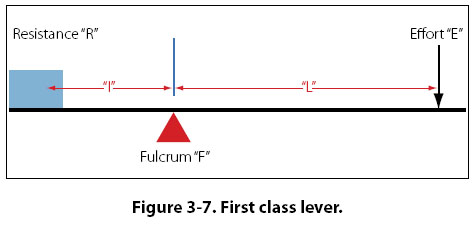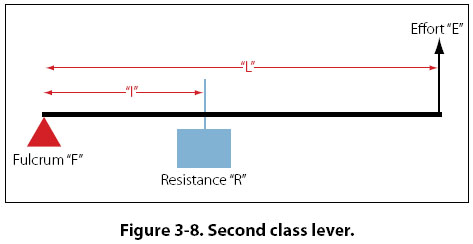The Lever The simplest machine, and perhaps the most familiar one, is the lever. A seesaw is a familiar example of a lever, with two people sitting on either end of a board and a pivoting point in the middle. There are three basic parts in all levers. They are the fulcrum “F," a force or effort “E," and a resistance “R." Shown in Figure 3-7 are the pivot point “F" (fulcrum), the effort “E" which is applied at a distance “L" from the fulcrum, and a resistance “R" which acts at a distance “l" from the fulcrum. Distances “L" and “l" are the lever arms.The concept of torque was discussed earlier in this chapter, and torque is very much involved in the operation of a lever. When a person sits on one end of a seesaw, that person applies a downward force in pounds which acts along the distance to the center of the seesaw. This combination of force and distance creates torque, which tries to cause rotation. First Class Lever In the first class lever, the fulcrum is located between the effort and the resistance. As mentioned earlier, the seesaw is a good example of a lever, and it happens to be a first class lever. The amount of weight and the distance from the fulcrum can be varied to suit the need. Increasing the distance from the applied effort to the fulcrum, compared to the distance from the fulcrum to the weight being moved, increases the advantage provided by the lever. Crowbars, shears, and pliers are common examples of this class of lever. The proper balance of an airplane is also a good example, with the center of lift on the wing being the pivot point (fulcrum) and the weight fore and aft of this point being the effort and the resistance. When calculating how much effort is required to lift a specific weight, or how much weight can be lifted by a specific effort, the following formula can be used. Effort (E) × Effort Arm (L) = Resistance (R) × Resistance Arm (l) What this formula really shows is the input torque (effort times effort arm) equals the output torque (resistance times resistance arm). This formula and concept apply to all three classes of levers, and to all simple machines in general. Example: A first class lever is to be used to lift a 500-lb weight. The distance from the weight to the fulcrum is 12 inches and from the fulcrum to the applied effort is 60 inches. How much force is required to lift the weight? Effort (E) × Effort Arm (L) = Resistance (R) × Resistance Arm (l) E × 60 in = 500 lb × 12 in E = 500 lb × 12 in ÷ 60 in E = 100 lb The mechanical advantage of the lever in this example would be: Mechanical Advantage = Force Out ÷ Force In = 500 lb ÷ 100 lb = 5, or 5 to 1 An interesting thing to note with this example lever is if the applied effort moved down 10 inches, the weight on the other end would only move up 2 inches. The weight being lifted would only move one-fifth as far. The reason for this is the concept of work. Because a lever cannot have more work output than input, if it allows you to lift 5 times more weight, you will only move it 1/5 as far as you move the effort. Second Class Lever The second class lever has the fulcrum at one end and the effort is applied at the other end. The resistance is somewhere between these points. A wheelbarrow is a good example of a second class lever, with the wheel at one end being the fulcrum, the handles at the opposite end being the applied effort, and the bucket in the middle being where the weight or resistance is placed. [Figure 3-8]Both first and second class levers are commonly used to help in overcoming big resistances with a relatively small effort. The first class lever, however, is more versatile. Depending on how close or how far away the weight is placed from the fulcrum, the first class lever can be made to gain force or gain distance, but not both at the same time. The second class lever can only be made to gain force. Example: The distance from the center of the wheel to the handles on a wheelbarrow is 60 inches. The weight in the bucket is 18 inches from the center of the wheel. If 300 lb is placed in the bucket, how much force must be applied at the handles to lift the wheelbarrow? Effort (E) × Effort Arm (L) = Resistance (R) × Resistance Arm (l) E × 60 inches = 300 lb × 18 in E = 300 lb × 18 in ÷ 60 in E = 90 lb The mechanical advantage of the lever in this example would be: Mechanical Advantage = Force Out ÷ Force In = 300 lb ÷ 90 lb = 3.33, or 3.33 to 1
 ©AvStop Online Magazine                                                                                                                                                      Contact Us              Return To Books